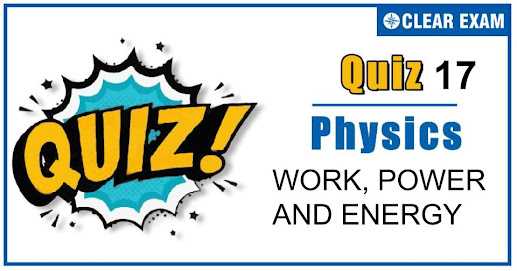## [LATEST]\$type=sticky\$show=home\$rm=0\$va=0\$count=4\$va=0

As per analysis for previous years, it has been observed that students preparing for NEET find Physics out of all the sections to be complex to handle and the majority of them are not able to comprehend the reason behind it. This problem arises especially because these aspirants appearing for the examination are more inclined to have a keen interest in Biology due to their medical background. Furthermore, sections such as Physics are dominantly based on theories, laws, numerical in comparison to a section of Biology which is more of fact-based, life sciences, and includes substantial explanations. By using the table given below, you easily and directly access to the topics and respective links of MCQs. Moreover, to make learning smooth and efficient, all the questions come with their supportive solutions to make utilization of time even more productive. Students will be covered for all their studies as the topics are available from basics to even the most advanced. .

Q1. Statement I In an elastic collision between two bodies, the relative speed of the bodies after collision is equal to the relative speed before the collision. Statement II In an elastic collision, the linear momentum of the system is conserved.?
•  Statement I is true ,statement II is true; statement II is a correct explanation for statement I
•  Statement I is true, Statement II is true; statement II is not correct explanation for statement I.
•  Statement I is true, Statement II is false .
•  Statement I is false, Statement II is True
Solution
In case of elastic collision ,coefficient of restitution e=1 or Relative speed of approach =relative speed of separation. ∴ Option (b) is correct.

Q2. A force acts on a 30 g particle in such a way that the position of the particle as a function of time is given by x=3t-4t2+t3, where x is in metres and t is in seconds. The work done during the first 4 seconds is?
•  5.28 J
•  450 mJ
•  190 mJ
•  530 mJ
Solution
v= dx/dt=3-8t+3t2 ∴v0=3 m/s and v4=19 m/s W=1/2 m(v42-v02) [According to work energy theorem] =1/2×0.03×(192-32 )=5.28 J

Q3. A ball is released from the top of a tower. The ratio of work done by force of gravity in first, second and third second of the motion of the ball is?
•  1:2:3
•  1:4:9
•  1:3:5
•  1:5:3
Solution
When the ball is released from the top of tower then ratio of distances covered by the ball in first, second and third second hI:hII:hIII=1:3:5: [Because hn∝(2n-1)] ∴ Ratio of work done mghI:mghII:mghIII=1:3:5

Q4. A spring with spring constant k is extended from x=0 to x=x1.The work done will be?
•  κx12
•  1/2 κx12
•  2κx12
•  2κx1
Solution
REPLACE DIAGRAM

Q5. A smooth sphere of mass M moving with velocity u directly collides elastically with another sphere of mass m at rest. After collision their final velocities are V and v respectively. The value of v is ?
•  2uM/m
•  2um/M
•  2u/(1+m/M)
•  2u/(1+M/m)
Q6. If a force F is applied on a body and it moves with a velocity v, the power will be?
•  F×v
•  F/v
•  F/v2
•  F×v2
Solution
F×v

Q7. The work done in pulling up a block of wood weighing 2 kN for a length of 10 m on a smooth plane inclined at an angle of 15° with the horizontal is [sin⁡15°=0.2588]?
•  4.36 kJ
•  5.17 kJ
•  8.91 kJ
•  9.82 kJ
Solution
W=mg sin⁡θ×s =2×103×sin⁡15°×10 =5.17 kJ

Q8. A wire is stretched under a force. If the wire suddenly snaps the temperature of the wire?
•  Remains the same
•  Decreases
•  Increases
•  First decreases then increases
Solution
Work done on the wire to strain it will be stored as energy which is converted to heat. Therefore the temperature increases

Q9. A mass of 100 g strikes the wall with speed 5 m/s at an angle as shown in figure and it rebounds with the same speed. If the contact time is 2×10-3 sec, what is the force applied on the mass by the wall?
•  250√3 N to right
•  250 N to right
•  250√3 N to left
•  250 N to left
Solution
Force = Rate of change of momentum Initial momentum P 1=mv sin⁡θ i ̂+mv cos⁡θ j ̂ Final momentum P 2=-mv sin⁡θ i ̂+mv cos⁡θ j ̂ ∴F =(∆P )/∆t=(-2mv sin⁡θ)/(2×10-3 ) Substituting m=0.1 kg,v=5 m/s,θ=60° Force on the ball F =-250√3 N Negative sign indicates direction of the force

Q10. A bullet of mass 0.02 kg travelling horizontally with velocity 250 ms-1 strikes a block of wood of mass 0.23 kg which rests on a rough horizontal surface. After the impact, the block and bullet move together and come to rest after travelling a distance of 40m. The coefficient of sliding friction of the rough surface is ( g=9.8 ms-2)?:
•  0.75
•  0.61
•  0.51
•  0.30
Solution
After impact the mass and block move together and come to rest after a distance of 40 m By conservation of momentum, m1 u1+m2 u2=m1 v1+m2 v2 0.02×250+0.23×0=0.02v+0.23v 5+0=v(0.25)
500/25=v=20 ms-1 Now, by conservation of energy, 1/2 Mv2=μR.d 1/2×0.25×400=μ×0.25×9.8×40⇒μ=0.51## Want to know more

Please fill in the details below:

## Latest NEET Articles\$type=three\$c=3\$author=hide\$comment=hide\$rm=hide\$date=hide\$snippet=hide

Name

ltr
item
BEST NEET COACHING CENTER | BEST IIT JEE COACHING INSTITUTE | BEST NEET & IIT JEE COACHING: Work,Power and Energy Quiz 17
Work,Power and Energy Quiz 17